### 6.3 Statistical Analysis of the Data

Many techniques have been proposed for statistically analysing fMRI data, and a variety of these are in general use. The aim of such analysis is to produce an image identifying the regions which show significant signal change in response to the task. Each pixel is assigned a value dependent on the likelihood of the null hypothesis, that the observed signal changes can be explained purely by random variation in the data consistent with its variance, being false. Such an image is called a statistical parametric map. The aim of this section is to show how such maps can be produced.

All the methods described below have been used, at one time or another, in the analysis of the data presented in this thesis. The majority have been implemented as 'C' programs, with the notable exception of using the SPM implementation of the general linear model.

Throughout this section, the analysis techniques described are demonstrated on an example data set. The experiment performed was intended to detect activations resulting from a visually cued motor task. The whole brain of the subject was imaged, in 16 coronal slices of resolution 3 x 3 x 10 mm3, every four seconds. As cued by an LED display, they were required to squeeze a ball at the rate of 2 Hz. The experiment involved 16 s of rest, followed by 16 s of task performance, repeated 32 times.

Further detail of the statistics mentioned in this chapter can be found in many statistics text books, for example those by Zar, and Miller and Freund.

#### 6.3.1 Subtraction Techniques

One of the simplest methods for obtaining results from a two state fMRI experiment is to perform a simple subtraction. This is carried out by averaging together all the images acquired during the 'on' phase of the task, and subtracting the average of all the 'off' images. The disadvantage of such a technique is that it is extremely sensitive to head motion, leading to large amounts of artefact in the image. Figure 6.7a shows a single slice through the motor cortex from the example data set, and Figure 6.7b shows the result of subtracting the 'off' images from the 'on' images. Although signal increase can be seen in the primary motor cortex, there is also a large amount of artefact, particularly at the boundaries in the image.

Such a method does not yield a statistic that can be tested against the null hypothesis, so instead of straight subtraction it is more common to use a Student's t-test. This weights the difference in means, by the standard deviation in 'off' or 'on' values, giving high t-scores to large differences with small standard deviations, and low t-scores to small differences with large standard deviations. The t-score is calculated on a pixel by pixel basis, for a time series X, using the formula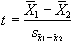(6.5)

where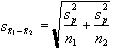(6.6)

and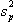is the pooled variance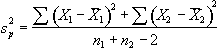. (6.7)

The suffix '1' refers to the n1 images acquired during the 'on' period of the task, and '2' refers to the n2 images acquired during the rest period. Figure 6.7c shows the statistical parametric map of t-scores for the sample data set. Again motor cortex activation is clearly seen, but the movement artefact is reduced compared to the subtraction technique.

(a)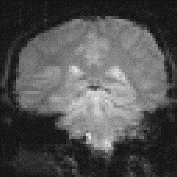(b)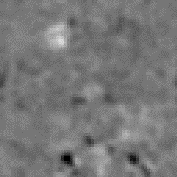(c)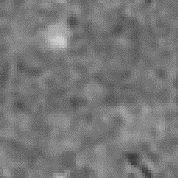#### 6.3.2 Correlation techniques

Since we know that the BOLD response is mediated by blood flow, it is possible to improve the detection of activations by predicting the shape of the response to the stimulus, and calculating correlation coefficients between each pixel time course and this reference waveform. This is less sensitive to other physiological changes during the experiment, and to movement. For a time course X, and a reference waveform Y, the correlation coefficient is calculated as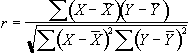, (6.8)

and has a value of 1 for perfect correlation, a value of zero for no correlation, and a value of -1 for perfect anti-correlation.

The choice of an appropriate reference waveform is vital for the success of this technique in finding activations. The first approximation might be a square wave, which is high for scans acquired during the task, and low for scans acquired during rest (Figure 6.8a). Such a waveform however takes no account of the delay and smoothness of the haemodynamic response which regulates the BOLD contrast. An improvement to this would be to change the phase of the square wave (Figure 6.8b), with the delay being between 3 and 6 seconds.

To improve the reference waveform further, it is necessary to look more closely at the actual haemodynamic response. In an experiment such as the one used for the example data set, where there is both visual and motor activation, it is possible to use the response to one type of stimulus to form the reference waveform for finding the other. In this case the time series for one or more pixels in, say the visual cortex is extracted (Figure 6.8c), and correlation coefficients are calculated between this waveform and that of every other pixel in the image. Such an analysis detects only those regions in the brain which respond to the stimulus in the same way as the visual cortex. The major disadvantage of this technique is that it is particularly sensitive to motion artefact, since if such artefact is present in the reference waveform then the movement of other regions will be highly correlated. To attempt to reduce this, the response in the visual cortex to each stimulus can be averaged together, producing a mean response to the single cycle. The reference waveform is then made up of a repetition of these single cycle average responses (Figure 6.8d).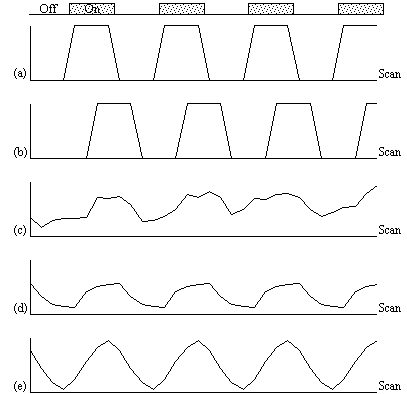##### Figure 6.8. Various reference functions that can be used to correlate with a pixel time course to detect activations (see text for descriptions)

To be more general in predicting the haemodynamic response, so that a reference waveform can be constructed for any length of stimulus, it is necessary to know the response to a single stimulus. Friston suggested that a haemodynamic response function could be considered as a point spread function, which smoothes and shifts the input function. By deconvolving the response from a known area of activation with the stimulus function, the haemodynamic response function can be obtained. The haemodynamic response function is not completely uniform across the entire brain however, and the shape obtained from one region may not be optimum for another. As an alternative, the response can be modelled by a mathematical function, such as a Poisson or beta function. The Poisson function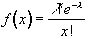(6.9)

with width l = 6 seconds, seems to fit well to observed haemodynamic responses (Figure 6.8e).

Since in general each slice of the volume imaged is not acquired at the same instant, it is necessary to accommodate timing differences in the correlation with the reference waveform. In order to do this, the relative magnitude of the activation at the time each slice was acquired is predicted, by convolving the input stimulus with a Poisson function. Then from this series, the correlation coefficients can be calculated on a slice by slice basis, constructing the reference waveform from the appropriate points in the predicted time series.

Examples of the effect of the reference waveform on the result is shown in Figure 6.9. Here, pixels in the head which correlate to the reference waveforms (shown in Figure 6.8), with r > 0.20 are shown in red, on top of the base image. The square wave correlation is the least effective in detecting activations (a), however a considerable improvement is obtained by delaying the waveform by 4 seconds (b). The correlation of the visual cortex with itself (c) is, not surprisingly, high, but using the average visual cortex response (d) improves the correlation in the motor cortex. The Poisson function model of the haemodynamic response (e) improves slightly on the delayed square wave, and is a good model.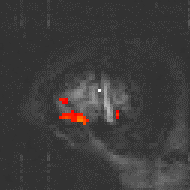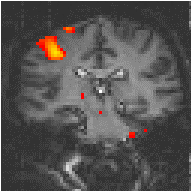(a) Square wave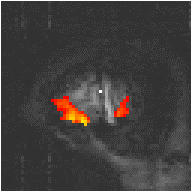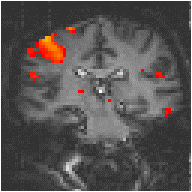(b) Delayed Square Wave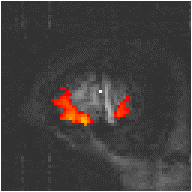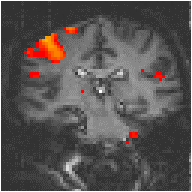(c) Visual Cortex Response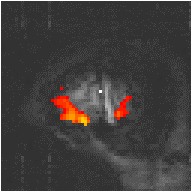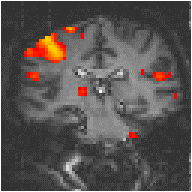(d) Average Visual Cortex Response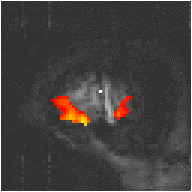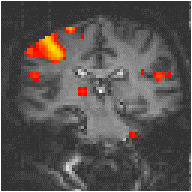(e) Poisson Distribution Model

#### 6.3.3 The General Linear Model

The statistical techniques described above are both parametric tests. That is to say, they assume that the observations are taken from normal populations. Most parametric modelling techniques are special cases of the general linear model. This framework for analysing functional imaging data, first developed for PET and then extended for fMRI, is implemented in the software package SPM. The general linear model is only outlined here, since the theory is extensively covered in the literature.

The aim of the general linear model is to explain the variation of the time course y1...yi...yn, in terms of a linear combination of explanatory variables and an error term. For a simple model with only one explanatory variable x1...xi...xn, the general linear model can be written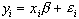(6.10)

where b is the scaling, or slope parameter, and ei is the error term. If the model includes more variables it is convenient to write the general linear model in matrix form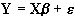, (6.11)

where now Y is the vector of observed pixel values, b is the vector of parameters and e is the vector of error terms. The matrix X is known as the design matrix. It has one row for every time point in the original data, and one column for every explanatory variable in the model. In analysing an fMRI experiment, the columns of X contain vectors corresponding to the 'on' and 'off' elements of the stimulus presented. By finding the magnitude of the parameter in b corresponding to these vectors, the presence or absence of activation can be detected.

b can be determined by solving the 'normal equations'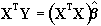(6.12)

where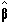is the best linear estimate of b. Provided that (XTX) is invertable thenis given by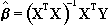. (6.13)

Such parameter estimates are normally distributed, and since the error term can be determined, statistical inference can be made as to whether the b parameter corresponding to the model of an activation response is significantly different from the null hypothesis.

The general linear model provides a framework for most kinds of modelling of the data, and can eliminate effects that may confound the analysis, such as drift or respiration, provided that they can be modelled.

#### 6.3.4 The Serial T-Test

All the techniques described above require the prediction of the time course that active regions will follow. For many experiments, the use of rapid imaging, and carefully designed paradigms, makes the separation of the order of cognitive events possible. One such example, which is part of our study of Parkinson's disease and described in more detail in Chapter 7, is a paradigm involving the initiation of movement. In this experiment, the subject was required to respond, by pressing a hand held button, to the visual presentation of the number '5', and to make no response to the presentation of a '2'. This paradigm presented two differences to conventional, epoch based experiments. Firstly, the activations of interest, which are those responsible for the button pressing, occurred at an irregular rate. Secondly, all the cognitive processes involved in the task, including both the planning and execution of the movement, occurred in a time period of a few hundred milliseconds, as opposed to the sustained activation used in epoch based paradigms. Such an experiment requires a new form of analysis. Two techniques have been evaluated, both of which make no assumptions about the time course of activations during the task: the serial t-test, described here, and an analysis of variance technique, explained in the next section.

The basis of the serial t-test is to define a resting state baseline, and compare the images acquired at each time point before, during and after the task with this baseline. Figure 6.10 illustrates the technique. For each time point following the stimulus, a mean and standard deviation image is constructed, as is a baseline mean and standard deviation image. Then a set of t-statistical parametric maps are formed by calculating, on a pixel by pixel basis, the t-score (using equations 6.5 - 6.7) for the difference between mean image one and the mean baseline image, mean image two and baseline, and so on.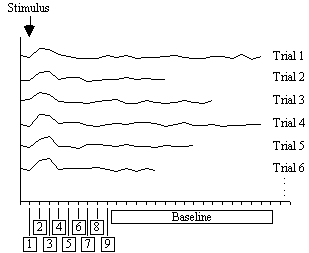##### Figure 6.10. fMRI analysis using the serial t-test

Figure 6.11 shows the result of analysing the example data set using this technique. Such a data set does not really show the benefit of the serial t-test analysis. Results shown in Chapter 7 better illustrate its use in looking at event timings, and unpredictable waveforms.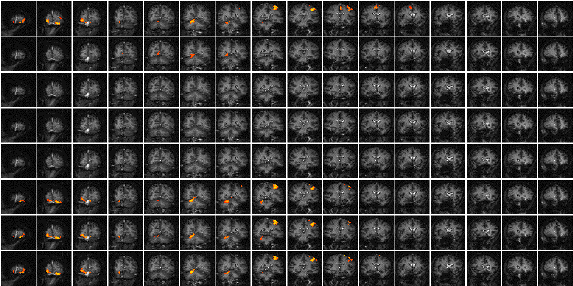##### Figure 6.11 Results of processing the test data set using the serial t-test. Eight volume image sets are shown as rows, the top four corresponding to the 'rest' periods of the experiment, and the next four corresponding to the 'task' periods. Activation can be seen in both the primary motor and visual cortices.

The technique has two major disadvantages. The first is that, in order to achieve a sufficient signal to noise ratio, it is necessary to have many more cycles than in an epoch based paradigm, thus leading to longer experiments. This can be uncomfortable for the subject, and puts additional demands on the scanner hardware. There is some scope for bringing the single event tasks closer together, but there must be a sufficient interval to allow the BOLD signal to return to baseline. This delay is at least ten seconds in length. The second disadvantage is that the analysis results in many statistical parametric maps, which have to be interpreted as a whole. However the fact that the technique makes few assumptions about the data time course makes it a strong technique, and opens up the possibility of more diverse experimental design, and a move away from the epoch based paradigms.

#### 6.3.5 Analysis of Variance

A second technique which does not require any assumptions about the shape of the activation time course, looks at the changes in variance upon averaging. The technique is based on simple signal averaging theory. Take, for example, the response measured to a repeated signal as shown in Figure 6.12. The time series contains two components, one is a genuine response to the signal, and the other is the random fluctuations due to uncorrelated physiological events and noise in the image. Upon averaging 32 cycles together, the magnitude of the noisy component is reduced but that of the repeated signal is not. The reduction of the noisy component can be measured by calculating the variance of both the unaveraged and averaged data set.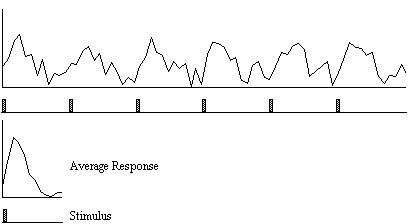##### Figure 6.12. Signal Averaging. The variance of the noise in the average signal is N times less than it is in the original signal, where N is the number of cycles.

To detect regions of activation, the ratio of the variance of the averaged data set to the variance of the unaveraged data set is calculated for each pixel in the image. For pixels in regions of purely random intensity variations, this ratio will be around 1/n, where n is the number of cycles averaged together. Pixels in regions of activation, however, will have a significantly higher ratio than this, since the variance of both unaveraged and averaged data sets is dominated by the stimulus locked intensity variations of the BOLD effect, which does not reduce upon averaging.

The technique is more formally explained as an analysis of variance (ANOVA). If Xij refers to the ith time point after the stimulus, of the jth cycle of an experiment

 time t X11, X12, ....., X1j, ....., X1n X1 time 2t X21, X22, ....., X2j, ....., X2n X2 ........ ..... ..... ..... ..... ..... ..... ..... time it Xi1, Xi2, ....., Xij, ....., Xin Xi ........ ..... ..... ..... ..... ..... ..... ..... time kt Xk1, Xk2, ....., Xkj, ....., Xkn Xk X

with n cycles and k points per cycle. The null hypothesis is that there is no significant difference in the means,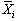. This can be tested by comparing two estimates of the population variance, s2, one based on variations in measurements of the same time point, and one based on the variance between time points.

The variance within measurement of any time point can be calculated by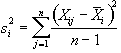, (6.14)

and so the mean variance within time points is given by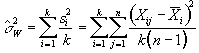, (6.15)

and is based on k(n-1) degrees of freedom. The variance of the time point means is given by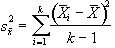, (6.16)

and since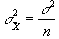(6.17)

then s2 can be estimated by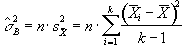(6.18)

which is based on k-1 degrees of freedom. Under the null hypothesis, both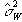and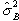independently estimate the population variance s2. This means that the ratio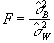(6.19)

will have an F distribution with k-1 and k(n-1) degrees of freedom. If there is any signal change that is time locked to the stimulus, the value ofwill be larger than expected under the null hypothesis.

In the analysis of fMRI data, equations 6.15 - 6.19 are used to form an F-statistical parametric map. These equations are implemented using the following short-cut formulas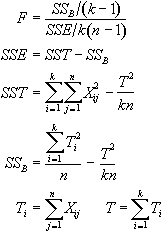(6.20)

To assess the validity of this approach on real data, phantom and head images were analysed. The phantom data set consisted of 256 volume images, each of 128 x 128 x 16 matrix size, obtained at a repetition rate of 4 seconds. The head data set consisted of 256 head images, of the same matrix size, with the subject performing no specified task. Both data sets were pre-processed in the same way that a functional imaging data set would be, and the analysis of variance was then carried out assuming 16 points per cycle and 16 cycles. Histograms of F for each data set are shown in Figure 6.13, with the appropriate F distribution, shown as a dotted line, given by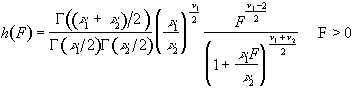(6.21)

where n1 is the number of points per cycle minus one, and n2 is the total number of data points minus the number of points per cycle. All three histograms show a good fit to the F distribution, confirming the validity of applying this technique to fMRI data.

 (a)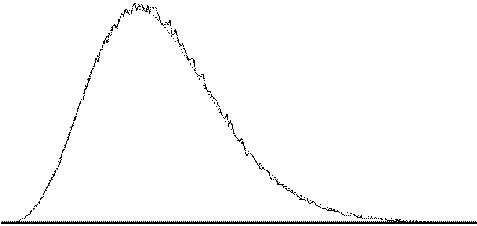(b)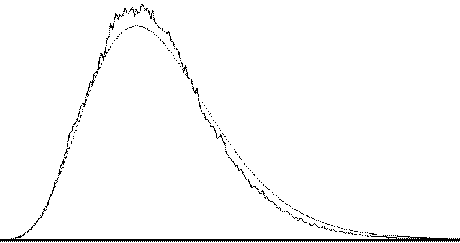(c)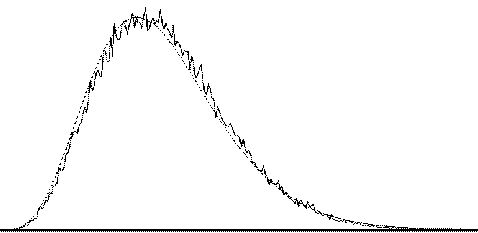##### Figure 6.13 Plots of calculated F-scores (solid line) and the appropriate F-distribution (dotted line) for (a) simulated data, (b) phantom data, and (c) head data.

The results of analysing the example activation data set using the ANOVA technique are shown in Figure 6.14. As with the serial t-test, such a data set does not best show the potential of this technique. A better example comes from the analysis of a study to investigate short term memory.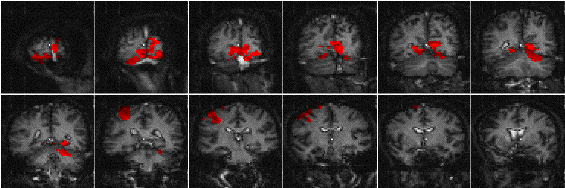##### Figure 6.14 Results of processing the test data set using the analysis of variance technique. Those pixels shaded in red correspond to regions which vary in some way that is time locked to the stimulus.

The stimulus paradigm for this experiment had three stages. First three digits in succession were presented to the subject. Eight seconds later a fourth digit was presented, and the subject was required to respond by pressing a button in their right hand if this final digit was the same as any of the three previously presented, or by pressing the button in their left hand if not. The final stage was a period of rest, to provide a baseline. The whole test was repeated 32 times.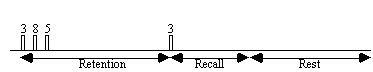It would be expected that some regions of the brain would only be active during the presentation of the digits, some during the retention period, some only in the recall stage and others throughout the whole memory task. To analyse such data using a correlation technique would mean predicting a whole set of reference waveforms. The ANOVA technique, however, detected the responses of different shapes in one test. Figure 6.15 shows the activation maps obtained from the ANOVA analysis of the short term memory experiment, together with time course plots from several of the areas.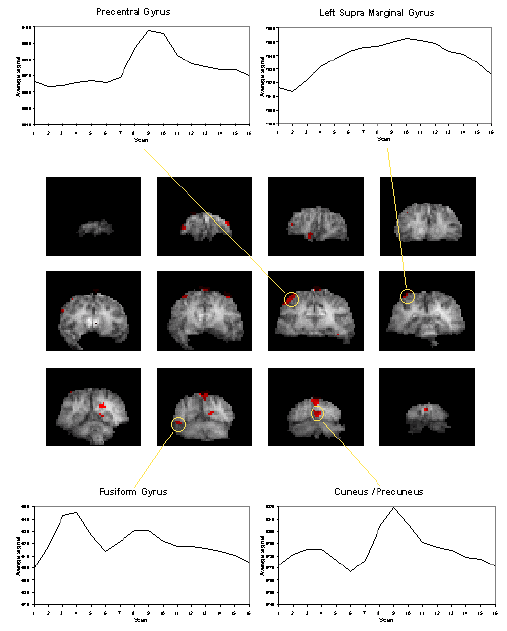##### Figure 6.15 Analysis of Variance technique applied to data from the short term memory experiment described in the text, together with average cycle plots for several regions of interest. Areas of the brain which act in different ways to the stimulus can be seen in a single activation image.

The final image of an ANOVA analysis, essentially shows all the regions which vary in some way that is synchronous to the presentation of the stimulus. It is equally good at picking up deactivations as activations. This makes these images a good starting point for other forms of analysis, such as principal component analysis or cluster analysis, so as to glean all the information that is available.

#### 6.3.6 Software Implementation

Due to the diversity of tests that can be carried out on one data set, software for implementing the tests described above was written as a set of separate programs.

The program correlate constructs a reference waveform, from user specified values, and optionally convolves this with a Poisson function of user specified width. Correlation coefficients are calculated using equation 6.8. If the reference waveform is made to vary between 0 and 1 then a measure of the percentage change upon activation can be obtained by calculating a linear regression of the form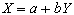where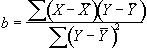(6.22)

and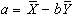.

The percentage change can be calculated as (b/a) x 100%.

The software also calculates the appropriate image of z-scores using Fishers Z transform and the reduced degrees of freedom as explained in the following sections. The file names for each of these outputs is

 cc_.img correlation coefficients saved as 'shorts' x 10,000 pc_.img percentage change saved as 'shorts' x 1,000 (such that 1% signal change x 1,000) cz_.img z-scores saved as 'floats' (not scaled)

The serial t-tests are performed by tmap and tmapnc, the first appropriate for cyclic experiments and the second for non-cyclic experiments. For the non-cyclic version the stimulus times are obtained from a text file, and both output t-score maps saved as 'shorts', scaled by 1000, in a file named tt_<file>.img.

ANOVA tests are carried out by the programs anova, and anovanc, which both output f-scores scaled by 1000, in a file named va_<file>.img.